Updated by Ashish Kumar - Let's Learn on Jan 27, 2022
REPORT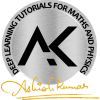12 items   2 followers   24 votes   2 views

# MathYug

Mathyug is an online education platform which provides Online Video Tutorials explained by expert teachers that will cover basics to advance level concepts, NCERT Solutions, R.D Sharma, R.S Aggarwal and Board’s Question Bank and Important Examples that one can access them directly to their desktop and mobile easily at any time and reach their educational goals by studying independently at your own pace.

1

## Full Syllabus of Class 12 Maths | Mathyug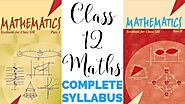Full Syllabus of Class 12 Maths having explanations from basic to advance concepts, each every NCERT solutions, questions, and important examples, NCERT Exemplar Problem, Board's Question Bank that are arranged within assignments created by Ashish Kumar (Agam Sir), which also include Notes in PDF form.

2

## Full Syllabus of Class 11 Maths | Mathyug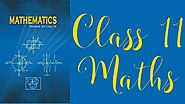Full syllabus of Class 11 Maths having explanation of all the 16 chapters, NCERT Exercise solutions, NCERT Examples, NCERT Exemplar Problem, Board's Question Bank that are arranged within assignments created by Ashish Kumar (Agam Sir), which also include Notes in PDF form.

3

## Basics of Limits and Derivatives Class 11 Maths Chapter 13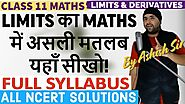High Quality Video Lecture explaining in detail about the basics and meaning of Limits and NCERT Solutions of Limits and Derivatives Class 11 Maths explained by expert teacher.

4

## Exercise 2.1 to 2.6 Units and Measurements Class 11 Physics IIT JEE Mains/NEET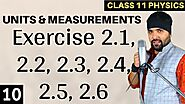High Quality Video Lecture explaining in detail about Units and Measurements and questions related to NCERT Exercise 2.1 to 2.6 of Chapter 2 Units and Measurements Class 11 Physics IIT JEE Mains/NEET explained by expert teacher.

5

## Exercise 3.4 to 3.7 Motion in a Straight Line Class 11 Physics IIT Jee Mains/ Neet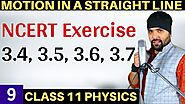Detailed explanation about Basics to advance concepts and questions related to NCERT Solutions for Exercise 3.4 to 3.7 of Chapter 3 Motion In A Straight Line Class 11 Physics explained by expert teacher.

6

## Questions based on Equivalence Relations Chapter 1 Relations and Functions Class 12 Maths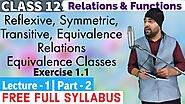Detailed explanation of Reflexive, Symmetric, Transitive, Equivalence Relations and NCERT Solutions for Exercise 1.1 Relations and Functions Class 12 Maths explained by expert teacher.

7

## NCERT Exercise 1.1 & 1.2 Chapter 1 Sets Class 11 Maths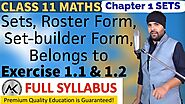High Quality Video Lecture explaining in detail about Sets, Roster Form, Set-builder form, and questions related to NCERT Exercise 1.1 & 1.2 of Sets Class 11 Maths explained by expert teacher.

8

## Basics of Logarithms, log table and antilog table | Class 11 Maths | Class 12 Maths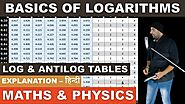Detailed explanation of meaning and basics of logarithms, exponents, how to read log table, how to read antilog table with the help of few examples for Class 11 Maths and Class 12 Maths.

9

## Lecture 1 (Part 1) - Chapter 1 Real Numbers Class 10 Maths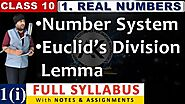Detailed explanation of Number System, Euclid's Division Lemma of NCERT Chapter 1 Real Numbers Class 10 Maths explained by expert teacher as per CBSE (NCERT) book guidelines.

10

## Basic Proportionality Theorem Chapter 6 Triangles Class 10 Maths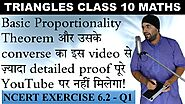Detailed explanation of Basic Proportionality Theorem and questions related to NCERT Solutions for Exercise 6.2 of Chapter 6 Triangles Class 10 Maths explained by expert teacher as per CBSE (NCERT) book guidelines.

11

## Three Dimensional Geometry Class 12 Maths IIT JEE Mains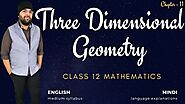Detailed explanation of NCERT Chapter 11 Three Dimensional Geometry Class 12 Maths Iit Jee Mains that will cover basics to advance level concepts, Ncert Solutions, R. D Sharma, R. S Aggarwal and Boards Question Bank and Important Examples.

12

## Number System and Euclid Division Lemma Chapter 10 Real Numbers Class 10 Maths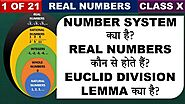Detailed explanation of Number System, Real Numbers and Euclid Division Lemma for NCERT Chapter 1 Real Numbers Class 10 Maths explained by expert teacher as per CBSE (NCERT) book guidelines.